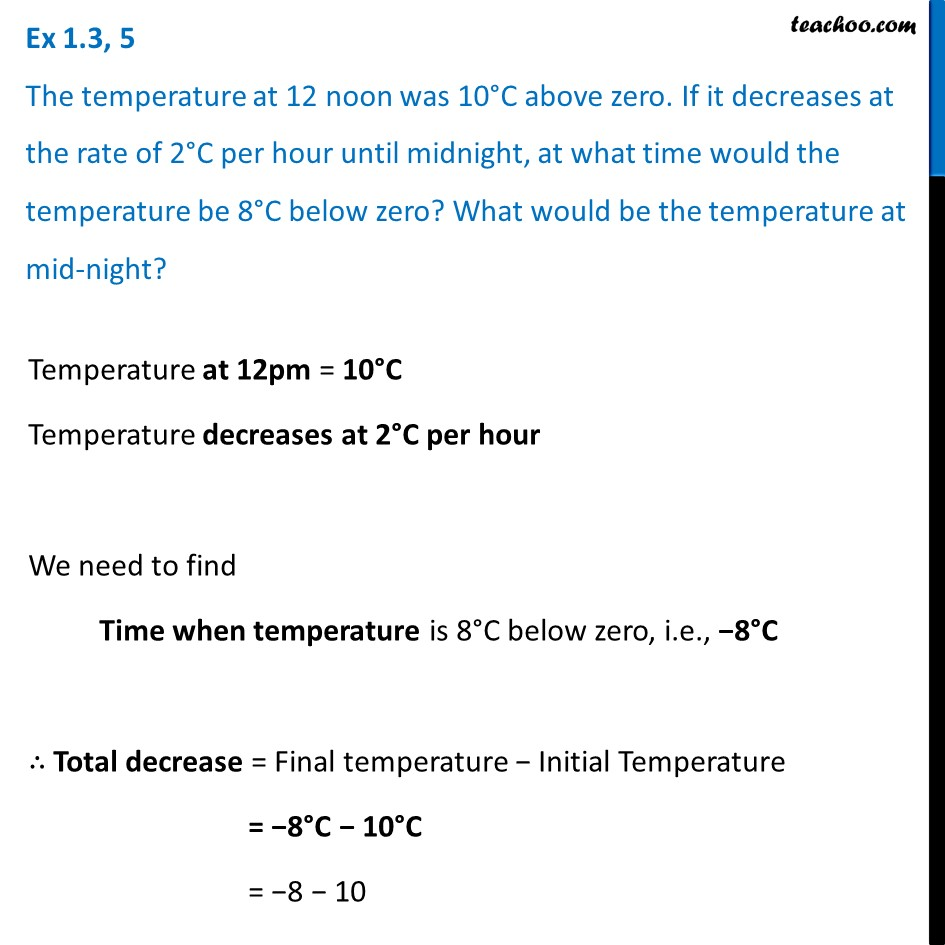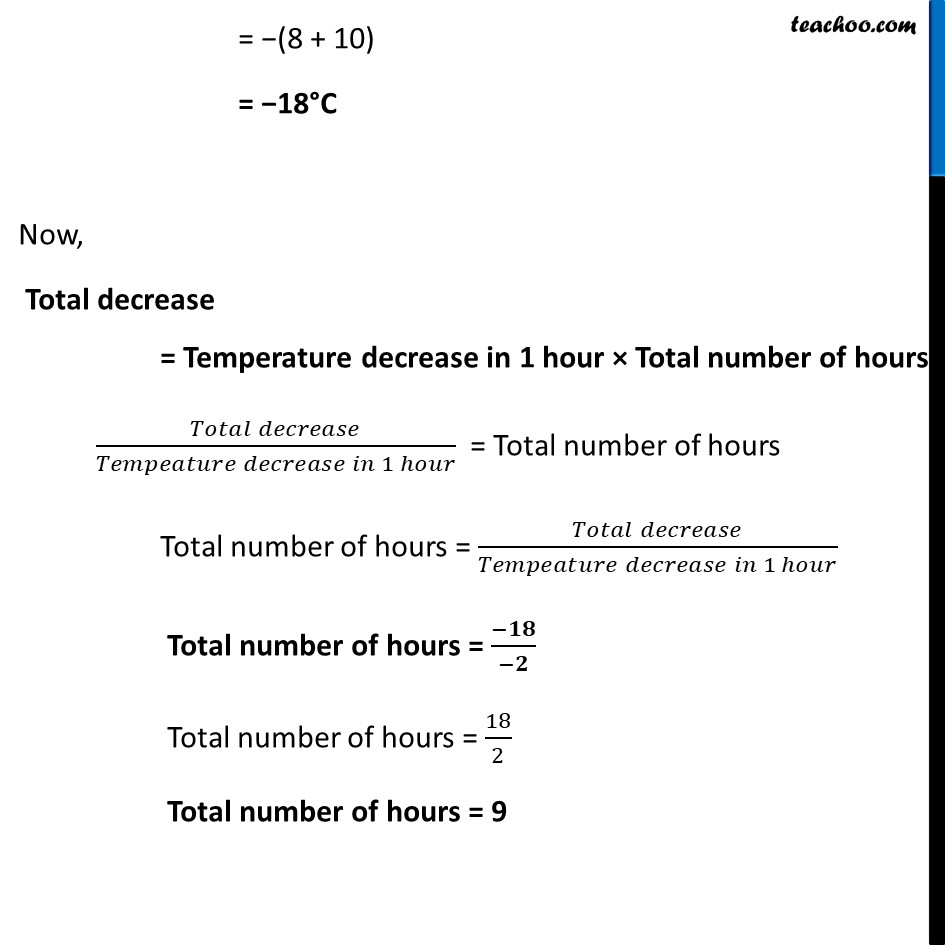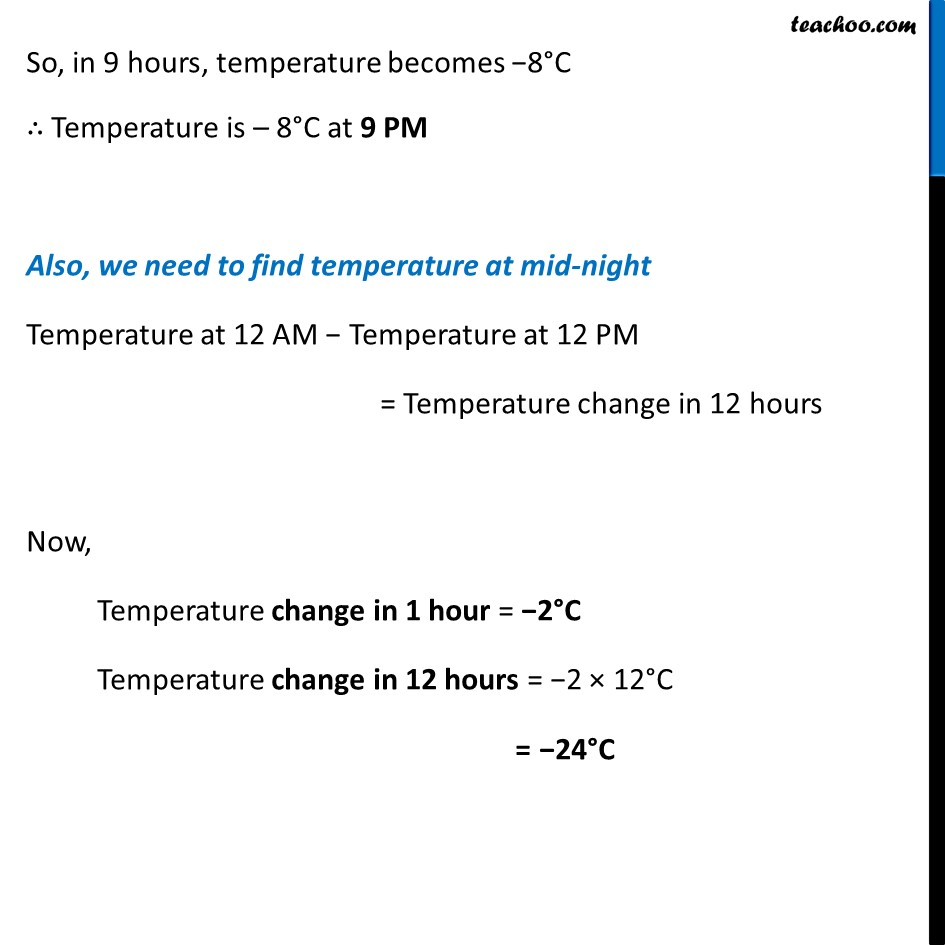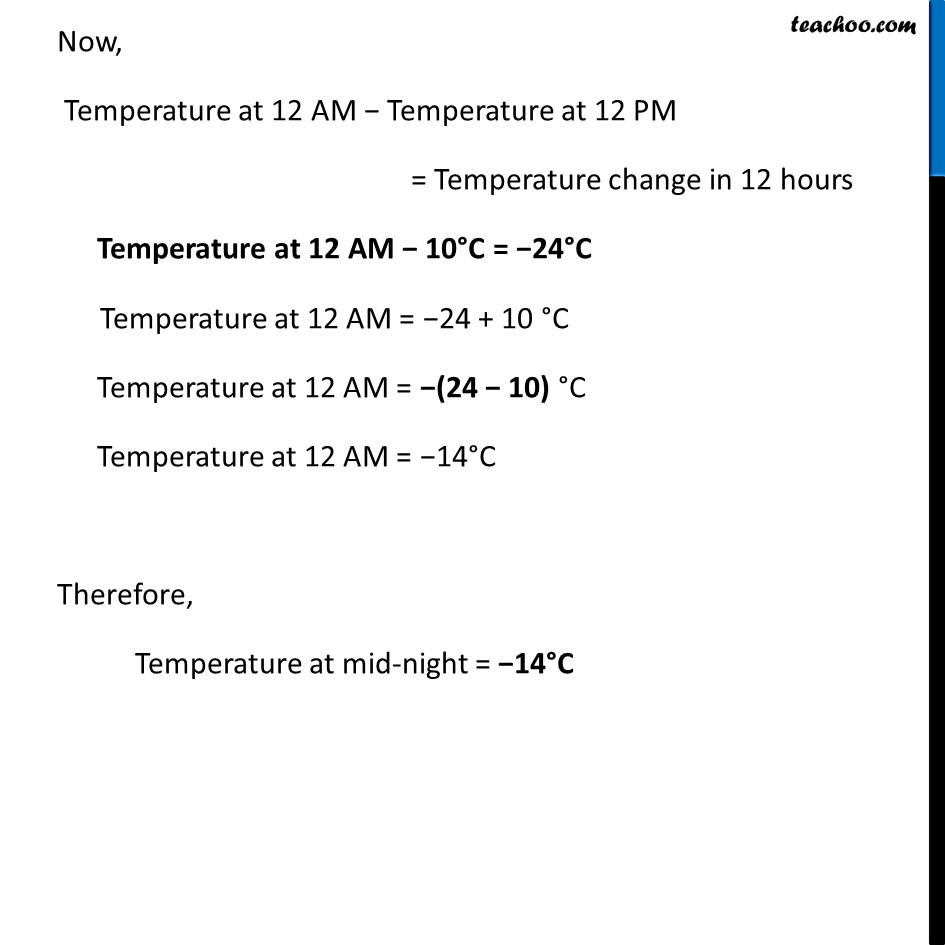Ex 1.3

Chapter 1 Class 7 Integers
Serial order wiseLearn in your speed, with individual attention - Teachoo Maths 1-on-1 Class

### Transcript

Ex 1.3, 5 The temperature at 12 noon was 10°C above zero. If it decreases at the rate of 2°C per hour until midnight, at what time would the temperature be 8°C below zero? What would be the temperature at mid-night?Temperature at 12pm = 10°C Temperature decreases at 2°C per hour We need to find Time when temperature is 8°C below zero, i.e., −8°C ∴ Total decrease = Final temperature − Initial Temperature = −8°C − 10°C = −8 − 10 = −(8 + 10) = −18°C Now, Total decrease = Temperature decrease in 1 hour × Total number of hours (𝑇𝑜𝑡𝑎𝑙 𝑑𝑒𝑐𝑟𝑒𝑎𝑠𝑒)/(𝑇𝑒𝑚𝑝𝑒𝑎𝑡𝑢𝑟𝑒 𝑑𝑒𝑐𝑟𝑒𝑎𝑠𝑒 𝑖𝑛 1 ℎ𝑜𝑢𝑟) = Total number of hours Total number of hours = (𝑇𝑜𝑡𝑎𝑙 𝑑𝑒𝑐𝑟𝑒𝑎𝑠𝑒)/(𝑇𝑒𝑚𝑝𝑒𝑎𝑡𝑢𝑟𝑒 𝑑𝑒𝑐𝑟𝑒𝑎𝑠𝑒 𝑖𝑛 1 ℎ𝑜𝑢𝑟) Total number of hours = (−𝟏𝟖)/(−𝟐) Total number of hours = 18/2 Total number of hours = 9 So, in 9 hours, temperature becomes −8°C ∴ Temperature is – 8°C at 9 PM Also, we need to find temperature at mid-night Temperature at 12 AM − Temperature at 12 PM = Temperature change in 12 hours Now, Temperature change in 1 hour = −2°C Temperature change in 12 hours = −2 × 12°C = −24°C Now, Temperature at 12 AM − Temperature at 12 PM = Temperature change in 12 hours Temperature at 12 AM − 10°C = −24°C Temperature at 12 AM = −24 + 10 °C Temperature at 12 AM = −(24 − 10) °C Temperature at 12 AM = −14°C Therefore, Temperature at mid-night = −14°C